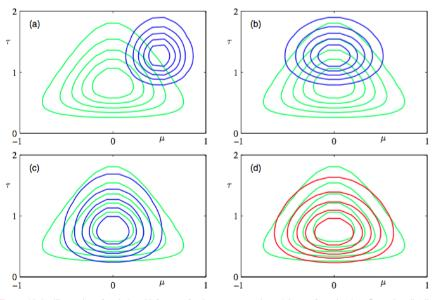Variational Bayesian Inference

2. Method

2.2 Bayes’ Theorem

P ( Z ∣ X ) = P ( X ∣ Z ) P ( Z ) P ( Z ) P(\textbf{Z}|\textbf{X})=\frac{P(\textbf{X}|\textbf{Z})P(\textbf{Z})}{P(\textbf{Z})}

2.3 Approximate Inference

P ( Z ∣ X ) ≈ Q ( Z ) P(\textbf{Z}|\textbf{X})\approx Q(\textbf{Z})2.4 Kullback-Leibler Divergence

D K L ( Q ( Z ) ∣ ∣ P ( Z ∣ X ) ) = ∑ Z Q ( Z ) log ⁡ Q ( Z ) P ( Z ∣ X ) D_{KL}\Big(Q(\textbf{Z})||P(\textbf{Z}|\textbf{X})\Big)=\sum_{\textbf{Z}}Q(\textbf{Z})\log \frac{Q(\textbf{Z})}{P(\textbf{Z}|\textbf{X})}

D K L ( Q ( Z ) ∣ ∣ P ( Z ∣ X ) ) = ∑ Z Q ( Z ) [ log ⁡ Q ( Z ) − log ⁡ P ( Z , X ) ] + ∑ Z Q ( Z ) [ log ⁡ P ( X ) ] D_{KL}\Big(Q(\textbf{Z})||P(\textbf{Z}|\textbf{X})\Big)=\sum_{\textbf{Z}}Q(\textbf{Z})\Big[\log Q(\textbf{Z})- \log P(\textbf{Z},\textbf{X})\Big]+\sum_{\textbf{Z}}Q(\textbf{Z})\Big[\log P(\textbf{X})\Big]

D K L ( Q ( Z ) ∣ ∣ P ( Z ∣ X ) ) = ∑ Z Q ( Z ) [ log ⁡ Q ( Z ) − log ⁡ P ( Z , X ) ] + log ⁡ P ( X ) D_{KL}\Big(Q(\textbf{Z})||P(\textbf{Z}|\textbf{X})\Big)=\sum_{\textbf{Z}}Q(\textbf{Z})\Big[\log Q(\textbf{Z})- \log P(\textbf{Z},\textbf{X})\Big]+\log P(\textbf{X})

log ⁡ P ( X ) = D K L ( Q ( Z ) ∣ ∣ P ( Z ∣ X ) ) + ∑ Z Q ( Z ) [ log ⁡ P ( Z , X ) − log ⁡ Q ( Z ) ] \log P(\textbf{X})= D_{KL}\Big(Q(\textbf{Z})||P(\textbf{Z}|\textbf{X})\Big)+\sum_{\textbf{Z}}Q(\textbf{Z})\Big[\log P(\textbf{Z},\textbf{X})-\log Q(\textbf{Z})\Big]

arg ⁡ min ⁡ Q D K L ( Q ( Z ) ∣ ∣ P ( Z ∣ X ) ) ↔ arg ⁡ max ⁡ Q ∑ Z Q ( Z ) [ log ⁡ P ( Z , X ) − log ⁡ Q ( Z ) ] \mathop{\arg \min}\limits_{Q} D_{KL}\Big(Q(\textbf{Z})||P(\textbf{Z}|\textbf{X})\Big)\leftrightarrow\mathop{\arg \max}\limits_{Q}\sum_{\textbf{Z}}Q(\textbf{Z})\Big[\log P(\textbf{Z},\textbf{X})-\log Q(\textbf{Z})\Big]

2.5 Mean field approximation

Q ( Z ) = ∏ i = m Q ( z i ) Q(\textbf{Z})=\prod_{i=}^{m}Q(z_i)

E L B O = ∑ Z ∏ i = 1 m Q ( z i ) [ log ⁡ P ( z i , X ) ] − ∑ Z ∏ i = 1 m Q ( z i ) [ log ⁡ Q ( z i ) ] ELBO=\sum_{\textbf{Z}}\prod_{i=1}^{m}Q(z_i)\Big[\log P(z_i,\textbf{X})\Big]-\sum_{\textbf{Z}}\prod_{i=1}^{m}Q(z_i)\Big[\log Q(z_i)\Big]

E L B O = ∑ Z ∏ i = 1 m Q ( z i ) [ log ⁡ P ( z i ∣ X ) ] + ∑ Z ∏ i = 1 m Q ( z i ) [ log ⁡ P ( X ) ] − ∑ Z ∏ i = 1 m Q ( z i ) [ log ⁡ Q ( z i ) ] ELBO=\sum_{\textbf{Z}}\prod_{i=1}^{m}Q(z_i)\Big[\log P(z_i|\textbf{X})\Big]+\sum_{\textbf{Z}}\prod_{i=1}^{m}Q(z_i)\Big[\log P(\textbf{X})\Big]-\sum_{\textbf{Z}}\prod_{i=1}^{m}Q(z_i)\Big[\log Q(z_i)\Big]

2.6 Black Box Variational Inference

E L B O = ∑ Z Q ( Z ; θ ) [ log ⁡ P ( Z , X ) − log ⁡ Q ( Z ; θ ) ] ELBO=\sum_{\textbf{Z}}Q(\textbf{Z};\theta)\Big[\log P(\textbf{Z},\textbf{X})-\log Q(\textbf{Z};\theta)\Big]

∇ E L B O = ∇ ∑ Z Q ( Z ; θ ) [ log ⁡ P ( Z , X ) Q ( Z ; θ ) ] \nabla ELBO=\nabla \sum_{\textbf{Z}} Q(\textbf{Z};\theta)\Big[\log \frac{P(\textbf{Z},\textbf{X})}{Q(\textbf{Z};\theta)}\Big]

▽ E L B O = ∑ Z ∇ Q ( Z ; θ ) [ log ⁡ P ( Z , X ) Q ( Z ; θ ) ] + ∑ Z Q ( Z ; θ ) ∇ [ log ⁡ P ( Z , X ) Q ( Z ; θ ) ] \triangledown ELBO=\sum_{\textbf{Z}} \nabla Q(\textbf{Z};\theta)\Big[\log \frac{P(\textbf{Z},\textbf{X})}{Q(\textbf{Z};\theta)}\Big] + \sum_{\textbf{Z}} Q(\textbf{Z};\theta)\nabla \Big[\log \frac{P(\textbf{Z},\textbf{X})}{Q(\textbf{Z};\theta)}\Big]

∇ E L B O = ∑ Z ∇ Q ( Z ; θ ) [ log ⁡ P ( Z , X ) Q ( Z ; θ ) ] \nabla ELBO=\sum_{\textbf{Z}} \nabla Q(\textbf{Z};\theta)\Big[\log \frac{P(\textbf{Z},\textbf{X})}{Q(\textbf{Z};\theta)}\Big]

∇ E L B O = ∑ Z Q ( Z ; θ ) ∇ Q ( Z ; θ ) Q ( Z ; θ ) [ log ⁡ P ( Z , X ) Q ( Z ; θ ) ] = ∑ Z Q ( Z ; θ ) ∇ [ ln ⁡ Q ( Z ; θ ) ] log ⁡ P ( Z , X ) Q ( Z ; θ ) \nabla ELBO=\sum_{\textbf{Z}}Q(\textbf{Z};\theta)\frac{\nabla Q(\textbf{Z};\theta)}{Q(\textbf{Z};\theta)}\Big[\log \frac{P(\textbf{Z},\textbf{X})}{Q(\textbf{Z};\theta)}\Big]=\sum_{\textbf{Z}}Q(\textbf{Z};\theta)\nabla\Big[\ln{Q(\textbf{Z};\theta)}\Big]\log \frac{P(\textbf{Z},\textbf{X})}{Q(\textbf{Z};\theta)}

∇ E L B O = 1 N ∑ i = 1 N F ( Z i ) = 1 N ∑ i = 1 N ∇ [ ln ⁡ Q ( Z i ; θ ) ] log ⁡ P ( Z i , X ) Q ( Z i ; θ ) \nabla ELBO = \frac1N \sum_{i=1}^N F(\textbf{Z}_i) = \frac1N \sum_{i=1}^N \nabla\Big[\ln{Q(\textbf{Z}_i;\theta)}\Big]\log \frac{P(\textbf{Z}_i,\textbf{X})}{Q(\textbf{Z}_i;\theta)}

θ t + 1 = θ t + η ∇ E L B O \theta^{t+1} = \theta^t + \eta \nabla ELBO

Reference

1. The on-line textbook: Information Theory, Inference, and Learning Algorithms, by David J.C. MacKay provides an introduction to variational methods (p. 422).
2. A Tutorial on Variational Bayes. Fox, C. and Roberts, S. 2012. Artificial Intelligence Review, doi:10.1007/s10462-011-9236-8.
3. Variational-Bayes Repository A repository of research papers, software, and links related to the use of variational methods for approximate Bayesian learning up to 2003.
4. Variational Algorithms for Approximate Bayesian Inference, by M. J. Beal includes comparisons of EM to Variational Bayesian EM and derivations of several models including Variational Bayesian HMMs.
5. High-Level Explanation of Variational Inference by Jason Eisner may be worth reading before a more mathematically detailed treatment.
6. Copula Variational Bayes inference via information geometry (pdf) by Tran, V.H. 2018. This paper is primarily written for students. Via Bregman divergence.
7. A Tutorial on Variational Bayesian InferenceCharles, Fox·Stephen Roberts
8. Variational Bayesian methods, Wikipedia
9. 如何简单易懂地理解变分推断(variational inference)？, 知乎
10. 一文学会变分推断及其求解方法, CSDN
11. 变分贝叶斯推断(Variational Bayes Inference)简介, CSDN
12. 变分推断（Variational Inference）最新进展简述, CSDN
13. 变分推断（Variational Inference）, 博客园
14. 机器学习, 周志华
本文为作者原创，转载请注明来源：https://blog.csdn.net/BrilliantAntonio/article/details/116885492